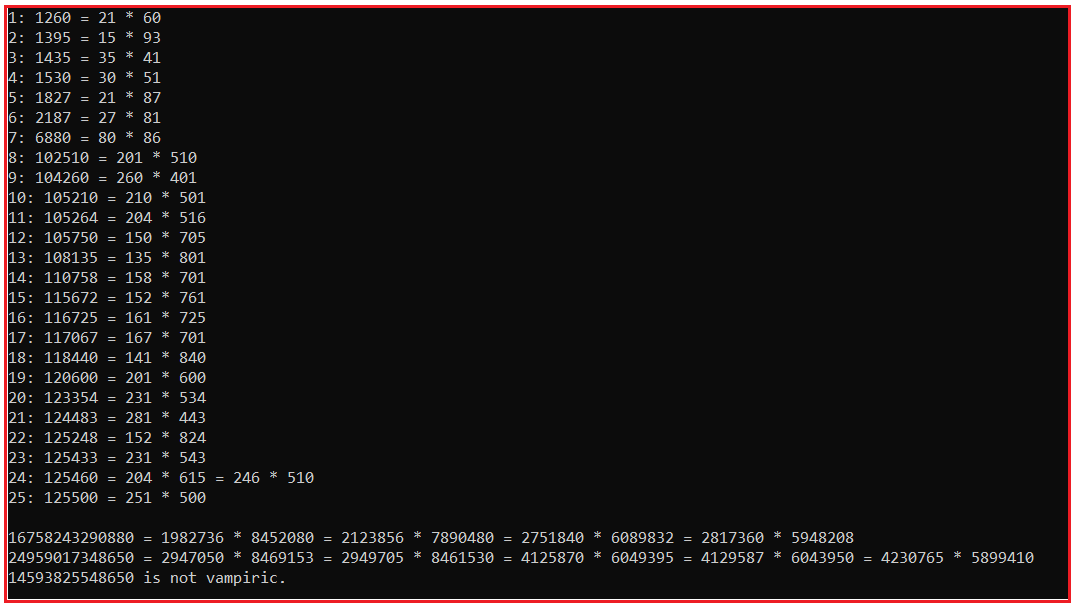# Vampire Number in C#

## Vampire Number in C# with Examples

In this article, I am going to discuss Vampire Number Program in C# with Examples. Please read our previous article where we discussed the Krishnamurthy Number Program in C#. Here, in this article, first, we will learn what is a Vampire Number and then we will see how to implement Vampire Number Program in C#.

##### What is a Vampire Number?

A vampire number is a natural number with an even number of digits, that can be factored into two integers. For example, 1260 is a vampire number, with 21 and 60 as fangs, since 21 × 60 = 1260.

126000 (which can be expressed as 21 × 6000 or 210 × 600) is not, as 21 and 6000 do not have the correct length, and both 210 and 600 have trailing zeroes

Some of the vampire numbers are:

1260, 1395, 1435, 1530, 1827, 2187, 6880, 102510, 104260, 105210, 105264, 105750, 108135, 110758, 115672, 116725, 117067, 118440, 120600, 123354, 124483, 125248, 125433, 125460, 125500, …

##### Multiple fang pairs

A vampire number can have multiple distinct pairs of fangs.
The first of infinitely many vampire numbers with 2 pairs of fangs: 125460 = 204 × 615 = 246 × 510
The first with 3 pairs of fangs: 13078260 = 1620 × 8073 = 1863 × 7020 = 2070 × 6318
The first with 4 pairs of fangs: 16758243290880 = 1982736 × 8452080 = 2123856 × 7890480 = 2751840 × 6089832 = 2817360 × 5948208

##### How to implement Vampire Number Program in C#?

In mathematics, a vampire number (or true vampire number) is a composite natural number v, with an even number of digits n, that can be factored into two integers x and y each with n/2 digits and not both with trailing zeroes, where v contains precisely all the digits from x and from y, in any order, counting multiplicity. x and y are called the fangs.

Condition for a number to be Vampire Number:

1. Has a pair number of digits. Let’s call the number of digits: n
2. You can obtain the number by multiplying two integers, x, and y, each with n/2 digits. x and y are the fangs.
3. Both fangs cannot end simultaneously in 0.
4. The number can be made with all digits from x and y, in any order and only using each digit once.
###### Example:

Positive natural number v = 1260
Even number of digits n = 4
Two integers x = 21 and y = 60 (Each integer contains n/2 i.e. 4/2 = 2 digits). There are no trailing zeros. Here, x and y are called fangs. Multiply x and y will give you 1260 and hence it is a Vampire Number

##### Example: Vampire Number Program in C#

The following sample code shows how to implement the Vampire number program in C#.

```using System;
namespace VampireNumber
{
class Program
{
static void Main(string[] args)
{
int i, j, n;
ulong x;
var f = new ulong;
var bigs = new ulong[] { 16758243290880UL, 24959017348650UL, 14593825548650UL, 0 };
ulong[] tens = new ulong;
tens = 1;
for (i = 1; i < 20; i++)
tens[i] = tens[i - 1] * 10;

for (x = 1, n = 0; n < 25; x++)
{
if ((j = fangs(x, f, tens)) == 0) continue;
Console.Write(++n + ": ");
show_fangs(x, f, j);
}

Console.WriteLine();
for (i = 0; bigs[i] > 0; i++)
{
if ((j = fangs(bigs[i], f, tens)) > 0)
show_fangs(bigs[i], f, j);
else
Console.WriteLine(bigs[i] + " is not vampiric.");
}
}

private static void show_fangs(ulong x, ulong[] f, int cnt)
{
Console.Write(x);
int i;
for (i = 0; i < cnt; i++)
Console.Write(" = " + f[i] + " * " + (x / f[i]));
Console.WriteLine();
}

private static int fangs(ulong x, ulong[] f, ulong[] tens)
{
int n = 0;
int nd = ndigits(x);
if ((nd & 1) > 0) return 0;
nd /= 2;

ulong lo, hi;
lo = Math.Max(tens[nd - 1], (x + tens[nd] - 2) / (tens[nd] - 1));
hi = Math.Min(x / lo, (ulong)Math.Sqrt(x));

ulong a, b, t = dtally(x);
for (a = lo; a <= hi; a++)
{
b = x / a;
if (a * b == x && ((a % 10) > 0 || (b % 10) > 0) && t == dtally(a) + dtally(b))
f[n++] = a;
}

return n;
}

private static ulong dtally(ulong x)
{
ulong t = 0;
while (x > 0)
{
t += 1UL << (int)((x % 10) * 6);
x /= 10;
}

return t;
}

private static int ndigits(ulong x)
{
int n = 0;
while (x > 0)
{
n++;
x /= 10;
}
return n;
}
}
}
```
###### Output:In the next article, I am going to discuss the Happy Number Program in C# with Examples. Here, in this article, I try to explain How to Implement Vampire Number Program in C# with Examples and I hope you enjoy this Vampire Number Program in the C# article.